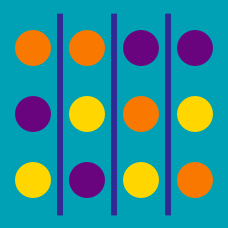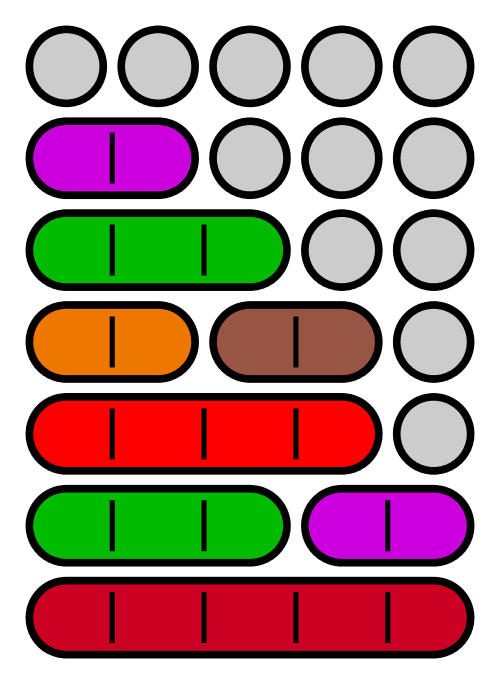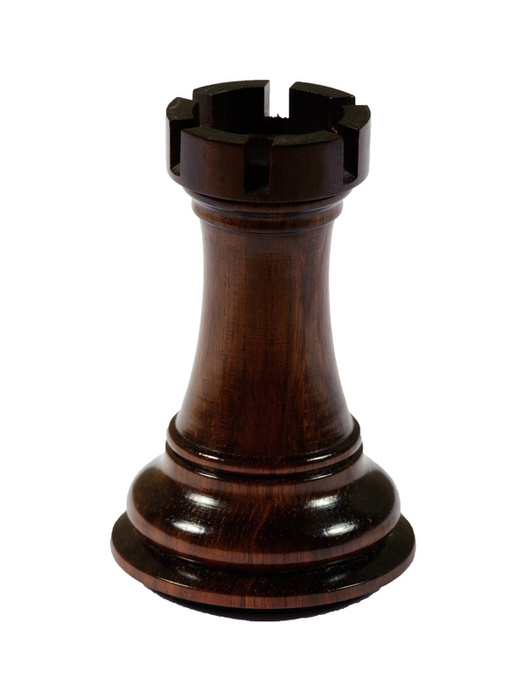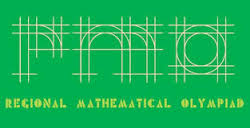Probability

# Permutations: Level 3 Challenges

How many positive integers have no repeated digits?The number of seven digit integers, with the sum of digits equal to 10 and formed by using the digits 1,2 and 3 only is,

How many 8 digit numbers are there, such that they are divisible by 9 and all of the digits are distinct?How many ways can you arrange 5 identical Rooks on a 6 by 10 Chessboard in such a way that none of the rooks attack each other ?Consider all the 7-digit numbers containing each of the digits 1, 2, 3, 4, 5, 6, 7 exactly once and that are not divisible by 5. They are arranged in a list in increasing order. Find the 2000th number in this list.

×